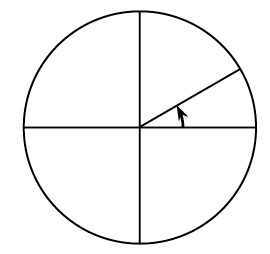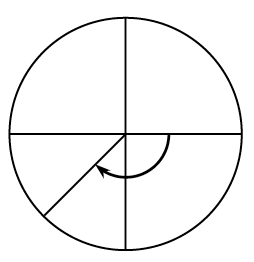### Home > PC3 > Chapter 2 > Lesson 2.1.2 > Problem2-23

2-23.

Below are two of the special angles that are used in the unit circle. Identify the radian measure for the angles shown. Remember counterclockwise angles are positive and clockwise angles are negative.

1.The top half of the circle is $\pi$ radians. How many equal parts can you divide the top half of the circle into using the given part?

1.The bottom half of the circle is $\pi$ radians. How many equal parts can you divide the bottom half of the circle into using the given part?

Three out of four pieces of $\pi$ radians are shaded.